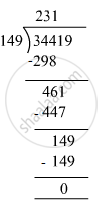# Divide and check your answer by the corresponding multiplication in the following: 34419 ​÷ 149 - Mathematics

Fill in the Blanks

Divide and check your answer by the corresponding multiplication in the following:

34419 ​÷ 149

#### SolutionDividend = 34419, Divisor = 149 , Quotient = 231, Remainder = 0
Check : Divisor × Quotient + Remainder = 149 × 231 + 0
= 34419
=Dividend
Hence, Dividend = Divisor × Quotient + Remainder

Concept: Concept for Whole Numbers
Is there an error in this question or solution?

#### APPEARS IN

RS Aggarwal Class 6 Mathematics
Chapter 3 Whole Numbers
Exercise 3E | Q 1.5 | Page 56